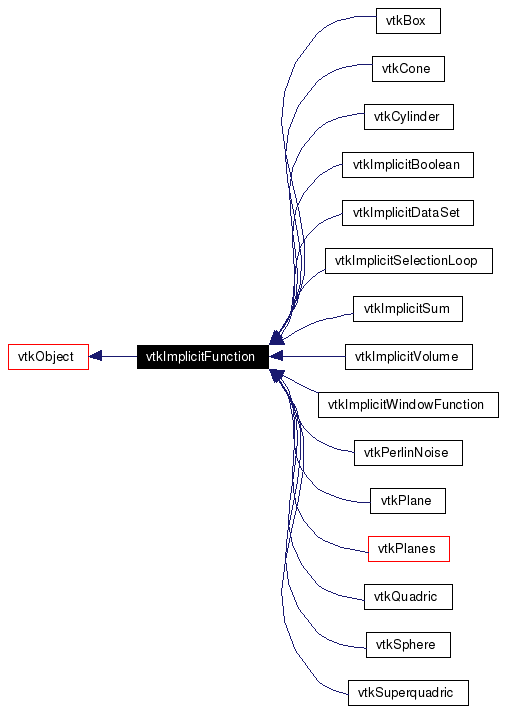# vtkImplicitFunction Class Reference

`#include <vtkImplicitFunction.h>`

Inheritance diagram for vtkImplicitFunction:[legend]
Collaboration diagram for vtkImplicitFunction:[legend]
List of all members.

## Detailed Description

abstract interface for implicit functions

vtkImplicitFunction specifies an abstract interface for implicit functions. Implicit functions are real valued functions defined in 3D space, w = F(x,y,z). Two primitive operations are required: the ability to evaluate the function, and the function gradient at a given point. The implicit function divides space into three regions: on the surface (F(x,y,z)=w), outside of the surface (F(x,y,z)>c), and inside the surface (F(x,y,z)<c). (When c is zero, positive values are outside, negative values are inside, and zero is on the surface. Note also that the function gradient points from inside to outside.)

Implicit functions are very powerful. It is possible to represent almost any type of geometry with the level sets w = const, especially if you use boolean combinations of implicit functions (see vtkImplicitBoolean).

vtkImplicitFunction provides a mechanism to transform the implicit function(s) via a vtkAbstractTransform. This capability can be used to translate, orient, scale, or warp implicit functions. For example, a sphere implicit function can be transformed into an oriented ellipse.

Warning:
The transformation transforms a point into the space of the implicit function (i.e., the model space). Typically we want to transform the implicit model into world coordinates. In this case the inverse of the transformation is required.
See also:
vtkAbstractTransform vtkSphere vtkCylinder vtkImplicitBoolean vtkPlane vtkPlanes vtkQuadric vtkImplicitVolume vtkSampleFunction vtkCutter vtkClipPolyData
Tests:
vtkImplicitFunction (Tests)

Definition at line 59 of file vtkImplicitFunction.h.

## Public Types

typedef vtkObject Superclass

## Public Member Functions

virtual const char * GetClassName ()
virtual int IsA (const char *type)
void PrintSelf (ostream &os, vtkIndent indent)
virtual void EvaluateGradient (double x, double g)=0
unsigned long GetMTime ()
double FunctionValue (const double x)
double FunctionValue (double x, double y, double z)
void FunctionGradient (const double x, double g)
double * FunctionGradient (const double x)
double * FunctionGradient (double x, double y, double z)
virtual void SetTransform (vtkAbstractTransform *)
virtual void SetTransform (double elements)
virtual vtkAbstractTransformGetTransform ()
virtual double EvaluateFunction (double x)=0
double EvaluateFunction (double x, double y, double z)

## Static Public Member Functions

static int IsTypeOf (const char *type)
static vtkImplicitFunctionSafeDownCast (vtkObject *o)

## Protected Member Functions

vtkImplicitFunction ()
~vtkImplicitFunction ()

## Protected Attributes

vtkAbstractTransformTransform
double ReturnValue 

## Member Typedef Documentation

 Reimplemented from vtkObject. Definition at line 62 of file vtkImplicitFunction.h.

## Constructor & Destructor Documentation

 vtkImplicitFunction::vtkImplicitFunction ( ) ` [protected]`

 vtkImplicitFunction::~vtkImplicitFunction ( ) ` [protected]`

## Member Function Documentation

 virtual const char* vtkImplicitFunction::GetClassName ( ) ` [virtual]`
 Reimplemented from vtkObject.

 static int vtkImplicitFunction::IsTypeOf ( const char * type ) ` [static]`
 Return 1 if this class type is the same type of (or a subclass of) the named class. Returns 0 otherwise. This method works in combination with vtkTypeRevisionMacro found in vtkSetGet.h. Reimplemented from vtkObject.

 virtual int vtkImplicitFunction::IsA ( const char * type ) ` [virtual]`
 Return 1 if this class is the same type of (or a subclass of) the named class. Returns 0 otherwise. This method works in combination with vtkTypeRevisionMacro found in vtkSetGet.h. Reimplemented from vtkObject.

 static vtkImplicitFunction* vtkImplicitFunction::SafeDownCast ( vtkObject * o ) ` [static]`
 Reimplemented from vtkObject.

 void vtkImplicitFunction::PrintSelf ( ostream & os, vtkIndent indent ) ` [virtual]`
 Methods invoked by print to print information about the object including superclasses. Typically not called by the user (use Print() instead) but used in the hierarchical print process to combine the output of several classes. Reimplemented from vtkObject.

 unsigned long vtkImplicitFunction::GetMTime ( ) ` [virtual]`
 Overload standard modified time function. If Transform is modified, then this object is modified as well. Reimplemented from vtkObject.

 double vtkImplicitFunction::FunctionValue ( const double x )
 Evaluate function at position x-y-z and return value. Point x is transformed through transform (if provided).

 double vtkImplicitFunction::FunctionValue ( double x, double y, double z ) ` [inline]`
 Overload standard modified time function. If Transform is modified, then this object is modified as well. Definition at line 73 of file vtkImplicitFunction.h.

 void vtkImplicitFunction::FunctionGradient ( const double x, double g )
 Evaluate function gradient at position x-y-z and pass back vector. Point x is transformed through transform (if provided).

 double* vtkImplicitFunction::FunctionGradient ( const double x ) ` [inline]`
 Evaluate function gradient at position x-y-z and pass back vector. Point x is transformed through transform (if provided). Definition at line 81 of file vtkImplicitFunction.h.

 double* vtkImplicitFunction::FunctionGradient ( double x, double y, double z ) ` [inline]`
 Evaluate function gradient at position x-y-z and pass back vector. Point x is transformed through transform (if provided). Definition at line 84 of file vtkImplicitFunction.h.

 virtual void vtkImplicitFunction::SetTransform ( vtkAbstractTransform * ) ` [virtual]`
 Set/Get a transformation to apply to input points before executing the implicit function.

 virtual void vtkImplicitFunction::SetTransform ( double elements ) ` [virtual]`
 Set/Get a transformation to apply to input points before executing the implicit function.

 virtual vtkAbstractTransform* vtkImplicitFunction::GetTransform ( ) ` [virtual]`
 Set/Get a transformation to apply to input points before executing the implicit function.

 virtual double vtkImplicitFunction::EvaluateFunction ( double x ) ` [pure virtual]`
 Evaluate function at position x-y-z and return value. You should generally not call this method directly, you should use FunctionValue() instead. This method must be implemented by any derived class.

 double vtkImplicitFunction::EvaluateFunction ( double x, double y, double z ) ` [inline]`
 Evaluate function at position x-y-z and return value. You should generally not call this method directly, you should use FunctionValue() instead. This method must be implemented by any derived class. Definition at line 102 of file vtkImplicitFunction.h.

 virtual void vtkImplicitFunction::EvaluateGradient ( double x, double g ) ` [pure virtual]`
 Evaluate function gradient at position x-y-z and pass back vector. You should generally not call this method directly, you should use FunctionGradient() instead. This method must be implemented by any derived class.

## Member Data Documentation

 vtkAbstractTransform* vtkImplicitFunction::Transform` [protected]`
 Definition at line 116 of file vtkImplicitFunction.h.

 double vtkImplicitFunction::ReturnValue` [protected]`
 Definition at line 117 of file vtkImplicitFunction.h.

The documentation for this class was generated from the following file:

Generated on Mon Jan 21 23:54:52 2008 for VTK by1.4.3-20050530